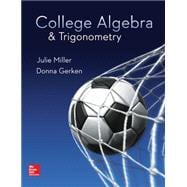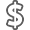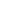# College Algebra & Trigonometry

• ISBN13:

• ISBN10:

## 0078035627

• Edition: 1st
• Format: Hardcover
• Publisher: McGraw-Hill Education

Note: Supplemental materials are not guaranteed with Rental or Used book purchases.

Purchase Benefits

•Free Shipping On Orders Over \$35!
Your order must be \$35 or more to qualify for free economy shipping. Bulk sales, PO's, Marketplace items, eBooks and apparel do not qualify for this offer.
•Get Rewarded for Ordering Your Textbooks! Enroll Now
•We Buy This Book Back!
In-Store Credit: \$86.17
Check/Direct Deposit: \$82.07
PayPal: \$82.07
List Price: \$264.60 Save up to \$231.85
• Rent Book \$32.75Free Shipping

TERM
PRICE
DUE
IN STOCK USUALLY SHIPS IN 24 HOURS.
HURRY! ONLY 2 COPIES IN STOCK AT THIS PRICE
*This item is part of an exclusive publisher rental program and requires an additional convenience fee. This fee will be reflected in the shopping cart.

### Supplemental Materials

What is included with this book?

• The New copy of this book will include any supplemental materials advertised. Please check the title of the book to determine if it should include any access cards, study guides, lab manuals, CDs, etc.
• The Used, Rental and eBook copies of this book are not guaranteed to include any supplemental materials. Typically, only the book itself is included. This is true even if the title states it includes any access cards, study guides, lab manuals, CDs, etc.

# College Algebra & Trigonometry © 2017

### Chapter R:Review of Prerequisites

SectionR.1Sets and the Real Number Line SectionR.2Integer Exponents and Scientific Notation SectionR.3Rational Exponents and Radicals SectionR.4Polynomials and Multiplication of Radicals Problem Recognition Exercises Simplifying Algebraic Expressions SectionR.5Factoring SectionR.6Rational Expressions and More Operations on Radicals Algebra for Calculus

### Chapter 1:Equations and Inequalities

Section1.1Linear Equations and Rational Equations Section1.2Applications with Linear and Rational Equations Section1.3Complex Numbers Section1.4Quadratic Equations Problem Recognition Exercises Simplifying Expressions Versus Solving Equations Section 1.5Applications of Quadratic Equations Section1.6More Equations and Applications Section1.7Linear, Compound, and Absolute Value Inequalities Problem Recognition Exercises Recognizing and Solving Equations and Inequalities Equations for Calculus

### Chapter 2:Functions and Relations

Section2.1The Rectangular Coordinate System and Graphing Utilities Section2.2Circles Section2.3Functions and Relations Section2.4Linear Equations in Two Variables and Linear Functions Section2.5Applications of Linear Equations and Modeling Problem Recognition Exercises Comparing Graphs of Equations Section2.6Transformations of Graphs Section2.7Analyzing Graphs of Functions and Piecewise-Defined Functions Section2.8Algebra of Functions and Function Composition

### Chapter 3:Polynomial and Rational Functions

Section3.1Quadratic Functions and Applications Section3.2Introduction to Polynomial Functions Section3.3Division of Polynomials and the Remainder and Factor Theorems Section3.4Zeros of Polynomials Section3.5Rational Functions Problem Recognition Exercises Polynomial and Rational Functions Section3.6Polynomial and Rational Inequalities Problem Recognition Exercises Solving Equations and Inequalities Section3.7Variation

### Chapter 4:Exponential and Logarithmic Functions

Section4.1Inverse Functions Section4.2Exponential Functions Section4.3Logarithmic Functions Problem Recognition Exercises Analyzing Functions Section4.4Properties of Logarithms Section4.5Exponential and Logarithmic Equations Section4.6Modeling with Exponential and Logarithmic Functions

### Chapter 5:Trigonometric Functions

Section5.1Angles and Their Measure Section5.2Right Triangle Trigonometry Section5.3Trigonometric Functions of Any Angle Section5.4Trigonometric Functions Defined on the Unit Circle Section5.5Graphs of Sine and Cosine Functions Section5.6Graphs of Other Trigonometric Functions Problem Recognition Exercises Comparing Graphical Characteristics of Trigonometric Functions Section5.7Inverse Trigonometric Functions

### Chapter 6:Analytic Trigonometry

Section6.1Fundamental Trigonometric Identities Section6.2Sum and Difference Formulas Section6.3Double-Angle and Half-Angle Formulas Section6.4Product-to-Sum and Sum-to-Product Formulas Section6.5Trigonometric Equations

### Chapter 7:Applications of Trigonometric Functions

Section7.1Applications of Right Triangles Section7.2The Law of Sines Section7.3The Law of Cosines Problem Recognition Exercises Solving Triangles Using a Variety of Tools Section7.4Harmonic Motion and Combinations of Trigonometric Functions

### Chapter 8:Trigonometry Applied to Rectangular and Polar Coordinate Systems and Vectors

Section8.1Polar Coordinates Section8.2Graphs of Polar Equations Problem Recognition Exercises Comparing Equations in Polar and Rectangular Form Section 8.3Complex Numbers in Polar Form Section8.4Vectors Section8.5Dot Product

### Chapter 9:Systems of Equations and Inequalities

Section9.1Systems of Linear Equations in Two Variables and Applications Section9.2Systems of Linear Equations in Three Variables and Applications Section9.3Partial Fraction Decomposition Section9.4Systems of Nonlinear Equations in Two Variables Section9.5Inequalities and Systems of Inequalities in Two Variables Problem Recognition Exercises Equations and Inequalities in Two Variables Section9.6Linear Programming

### Chapter 10:Matrices and Determinants and Applications

Section10.1Solving Systems of Linear Equations Using Matrices Section10.2Inconsistent Systems and Dependent Equations Section10.3Operations on Matrices Section10.4Inverse Matrices and Matrix Equations Section10.5Determinants and Cramer's Rule Problem Recognition Exercises Using Multiple Methods to Solve Systems of Linear Equations

### Chapter 11:Analytic Geometry

Section11.1The Ellipse Section11.2The Hyperbola Section11.3The Parabola Problem Recognition Exercises Comparing Equations of Conic Sections and General Equation Section11.4Rotation of Axes Section11.5Polar Equations of Conics Section11.6Plane Curves and Parametric Equations

### Chapter 12:Sequences, Series, Induction, and Probability

Section12.1Sequences and Series Section12.2Arithmetic Sequences and Series Section12.3Geometric Sequences and Series Problem Recognition Exercises Comparing Arithmetic and Geometric Sequences and Series Section12.4Mathematical Induction Section12.5The Binomial Theorem Section12.6Principles of Counting Section12.7Introduction to Probability